Main Content

# plotPartialDependence

Create partial dependence plot (PDP) and individual conditional expectation (ICE) plots

## Syntax

``plotPartialDependence(RegressionMdl,Vars)``
``plotPartialDependence(ClassificationMdl,Vars,Labels)``
``plotPartialDependence(___,Data)``
``plotPartialDependence(___,Name,Value)``
``ax = plotPartialDependence(___)``

## Description

example

````plotPartialDependence(RegressionMdl,Vars)` computes and plots the partial dependence between the predictor variables listed in `Vars` and the responses predicted by using the regression model `RegressionMdl`, which contains predictor data. If you specify one variable in `Vars`, the function creates a line plot of the partial dependence against the variable.If you specify two variables in `Vars`, the function creates a surface plot of the partial dependence against the two variables. ```

example

````plotPartialDependence(ClassificationMdl,Vars,Labels)` computes and plots the partial dependence between the predictor variables listed in `Vars` and the scores for the classes specified in `Labels` by using the classification model `ClassificationMdl`, which contains predictor data. If you specify one variable in `Vars` and one class in `Labels`, the function creates a line plot of the partial dependence against the variable for the specified class.If you specify one variable in `Vars` and multiple classes in `Labels`, the function creates a line plot for each class on one figure.If you specify two variables in `Vars` and one class in `Labels`, the function creates a surface plot of the partial dependence against the two variables. ```

example

````plotPartialDependence(___,Data)` uses new predictor data `Data`. You can specify `Data` in addition to any of the input argument combinations in the previous syntaxes.```

example

````plotPartialDependence(___,Name,Value)` uses additional options specified by one or more name-value pair arguments. For example, if you specify `'Conditional','absolute'`, the `plotPartialDependence` function creates a figure including a PDP, a scatter plot of the selected predictor variable and predicted responses or scores, and an ICE plot for each observation.```

example

````ax = plotPartialDependence(___)` returns the axes of the plot.```

## Examples

collapse all

Train a regression tree using the `carsmall` data set, and create a PDP that shows the relationship between a feature and the predicted responses in the trained regression tree.

Load the `carsmall` data set.

`load carsmall`

Specify `Weight`, `Cylinders`, and `Horsepower` as the predictor variables (`X`), and `MPG` as the response variable (`Y`).

```X = [Weight,Cylinders,Horsepower]; Y = MPG;```

Train a regression tree using `X` and `Y`.

`Mdl = fitrtree(X,Y);`

View a graphical display of the trained regression tree.

`view(Mdl,'Mode','graph')`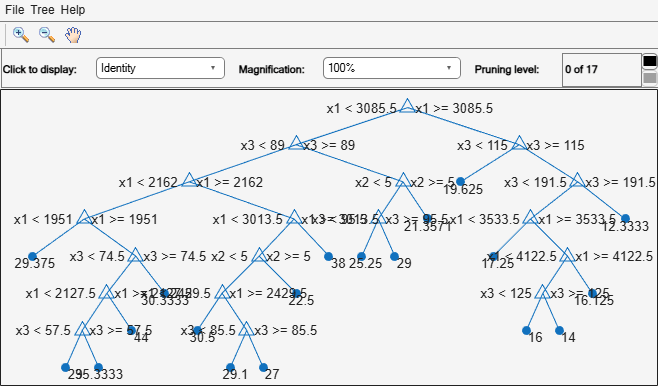Create a PDP of the first predictor variable, `Weight`.

`plotPartialDependence(Mdl,1)`The plotted line represents averaged partial relationships between `Weight` (labeled as `x1`) and `MPG` (labeled as `Y`) in the trained regression tree `Mdl`. The `x`-axis minor ticks represent the unique values in `x1`.

The regression tree viewer shows that the first decision is whether `x1` is smaller than 3085.5. The PDP also shows a large change near `x1` = 3085.5. The tree viewer visualizes each decision at each node based on predictor variables. You can find several nodes split based on the values of `x1`, but determining the dependence of `Y` on `x1` is not easy. However, the `plotPartialDependence` plots average predicted responses against `x1`, so you can clearly see the partial dependence of `Y` on `x1`.

The labels `x1` and `Y` are the default values of the predictor names and the response name. You can modify these names by specifying the name-value pair arguments `'PredictorNames'` and `'ResponseName'` when you train `Mdl` using `fitrtree`. You can also modify axis labels by using the `xlabel` and `ylabel` functions.

Train a naive Bayes classification model with the `fisheriris` data set, and create a PDP that shows the relationship between the predictor variable and the predicted scores (posterior probabilities) for multiple classes.

Load the `fisheriris` data set, which contains species (`species`) and measurements (`meas`) on sepal length, sepal width, petal length, and petal width for 150 iris specimens. The data set contains 50 specimens from each of three species: setosa, versicolor, and virginica.

`load fisheriris`

Train a naive Bayes classification model with `species` as the response and `meas` as predictors.

`Mdl = fitcnb(meas,species);`

Create a PDP of the scores predicted by `Mdl` for all three classes of `species` against the third predictor variable `x3`. Specify the class labels by using the `ClassNames` property of `Mdl`.

`plotPartialDependence(Mdl,3,Mdl.ClassNames);`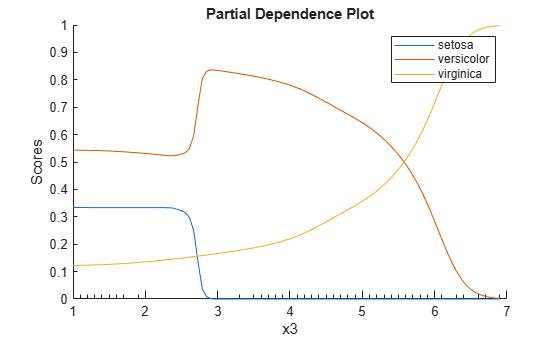According to this model, the probability of `virginica` increases with `x3`. The probability of `setosa` is about 0.33, from where `x3` is 0 to around 2.5, and then the probability drops to almost 0.

Train a Gaussian process regression model using generated sample data where a response variable includes interactions between predictor variables. Then, create ICE plots that show the relationship between a feature and the predicted responses for each observation.

Generate sample predictor data `x1` and `x2`.

```rng('default') % For reproducibility n = 200; x1 = rand(n,1)*2-1; x2 = rand(n,1)*2-1;```

Generate response values that include interactions between `x1` and `x2`.

`Y = x1-2*x1.*(x2>0)+0.1*rand(n,1);`

Create a Gaussian process regression model using `[x1 x2]` and `Y`.

`Mdl = fitrgp([x1 x2],Y);`

Create a figure including a PDP (red line) for the first predictor `x1`, a scatter plot (circle markers) of `x1` and predicted responses, and a set of ICE plots (gray lines) by specifying `'Conditional'` as `'centered'`.

`plotPartialDependence(Mdl,1,'Conditional','centered')`When `'Conditional'` is `'centered'`, `plotPartialDependence` offsets plots so that all plots start from zero, which is helpful in examining the cumulative effect of the selected feature.

A PDP finds averaged relationships, so it does not reveal hidden dependencies especially when responses include interactions between features. However, the ICE plots clearly show two different dependencies of responses on `x1`.

Train an ensemble of classification models and create two PDPs, one using the training data set and the other using a new data set.

Load the `census1994` data set, which contains US yearly salary data, categorized as `<=50K` or `>50K`, and several demographic variables.

`load census1994`

Extract a subset of variables to analyze from the tables `adultdata` and `adulttest`.

```X = adultdata(:,{'age','workClass','education_num','marital_status','race', ... 'sex','capital_gain','capital_loss','hours_per_week','salary'}); Xnew = adulttest(:,{'age','workClass','education_num','marital_status','race', ... 'sex','capital_gain','capital_loss','hours_per_week','salary'});```

Train an ensemble of classifiers with `salary` as the response and the remaining variables as predictors by using the function `fitcensemble`. For binary classification, `fitcensemble` aggregates 100 classification trees using the `LogitBoost` method.

`Mdl = fitcensemble(X,'salary');`

Inspect the class names in `Mdl`.

`Mdl.ClassNames`
```ans = 2x1 categorical <=50K >50K ```

Create a partial dependence plot of the scores predicted by `Mdl` for the second class of `salary` (`>50K`) against the predictor `age` using the training data.

`plotPartialDependence(Mdl,'age',Mdl.ClassNames(2))`Create a PDP of the scores for class `>50K` against `age` using new predictor data from the table `Xnew`.

`plotPartialDependence(Mdl,'age',Mdl.ClassNames(2),Xnew)`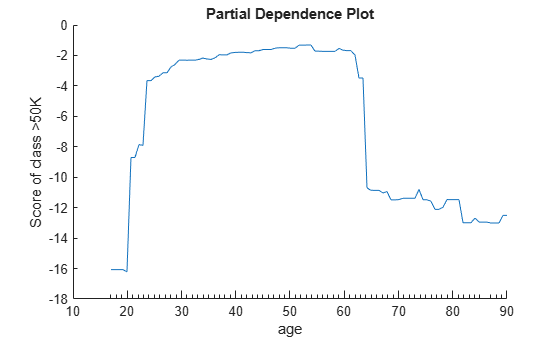The two plots show similar shapes for the partial dependence of the predicted score of high `salary` (`>50K`) on `age`. Both plots indicate that the predicted score of high salary rises fast until the age of 30, then stays almost flat until the age of 60, and then drops fast. However, the plot based on the new data produces slightly higher scores for ages over 65.

Train a regression ensemble using the `carsmall` data set, and create a PDP plot and ICE plots for each predictor variable using a new data set, `carbig`. Then, compare the figures to analyze the importance of predictor variables. Also, compare the results with the estimates of predictor importance returned by the `predictorImportance` function.

Load the `carsmall` data set.

`load carsmall`

Specify `Weight`, `Cylinders`, `Horsepower`, and `Model_Year` as the predictor variables (`X`), and `MPG` as the response variable (`Y`).

```X = [Weight,Cylinders,Horsepower,Model_Year]; Y = MPG;```

Train a regression ensemble using `X` and `Y`.

```Mdl = fitrensemble(X,Y, ... 'PredictorNames',{'Weight','Cylinders','Horsepower','Model Year'}, ... 'ResponseName','MPG');```

Create the importance of predictor variables by using the `plotPartialDependence` and `predictorImportance` functions. The `plotPartialDependence` function visualizes the relationships between a selected predictor and predicted responses. `predictorImportance` summarizes the importance of a predictor with a single value.

Create a figure including a PDP plot (red line) and ICE plots (gray lines) for each predictor by using `plotPartialDependence` and specifying `'Conditional','absolute'`. Each figure also includes a scatter plot (circle markers) of the selected predictor and predicted responses. Also, load the `carbig` data set and use it as new predictor data, `Xnew`. When you provide `Xnew`, the `plotPartialDependence` function uses `Xnew` instead of the predictor data in `Mdl`.

```load carbig Xnew = [Weight,Cylinders,Horsepower,Model_Year]; figure t = tiledlayout(2,2,'TileSpacing','compact'); title(t,'Individual Conditional Expectation Plots') for i = 1 : 4 nexttile plotPartialDependence(Mdl,i,Xnew,'Conditional','absolute') title('') end```Compute estimates of predictor importance by using `predictorImportance`. This function sums changes in the mean squared error (MSE) due to splits on every predictor, and then divides the sum by the number of branch nodes.

```imp = predictorImportance(Mdl); figure bar(imp) title('Predictor Importance Estimates') ylabel('Estimates') xlabel('Predictors') ax = gca; ax.XTickLabel = Mdl.PredictorNames;```The variable `Weight` has the most impact on `MPG` according to predictor importance. The PDP of `Weight` also shows that `MPG` has high partial dependence on `Weight`. The variable `Cylinders` has the least impact on `MPG` according to predictor importance. The PDP of `Cylinders` also shows that `MPG` does not change much depending on `Cylinders`.

Train a generalized additive model (GAM) with both linear and interaction terms for predictors. Then, create a PDP with both linear and interaction terms and a PDP with only linear terms. Specify whether to include interaction terms when creating the PDPs.

Load the `ionosphere` data set. This data set has 34 predictors and 351 binary responses for radar returns, either bad (`'b'`) or good (`'g'`).

`load ionosphere`

Train a GAM using the predictors `X` and class labels `Y`. A recommended practice is to specify the class names. Specify to include the 10 most important interaction terms.

`Mdl = fitcgam(X,Y,'ClassNames',{'b','g'},'Interactions',10);`

`Mdl` is a `ClassificationGAM` model object.

List the interaction terms in `Mdl`.

`Mdl.Interactions`
```ans = 10×2 1 5 7 8 6 7 5 6 5 7 5 8 3 5 4 7 1 7 4 5 ```

Each row of `Interactions` represents one interaction term and contains the column indexes of the predictor variables for the interaction term.

Find the most frequent predictor in the interaction terms.

`mode(Mdl.Interactions,'all')`
```ans = 5 ```

The most frequent predictor in the interaction terms is the 5th predictor (`x5`). Create PDPs for the 5th predictor. To exclude interaction terms from the computation, specify `'IncludeInteractions',false` for the second PDP.

```plotPartialDependence(Mdl,5,Mdl.ClassNames(1)) hold on plotPartialDependence(Mdl,5,Mdl.ClassNames(1),'IncludeInteractions',false) grid on legend('Linear and interaction terms','Linear terms only') title('PDPs of Posterior Probabilities for 5th Predictor') hold off```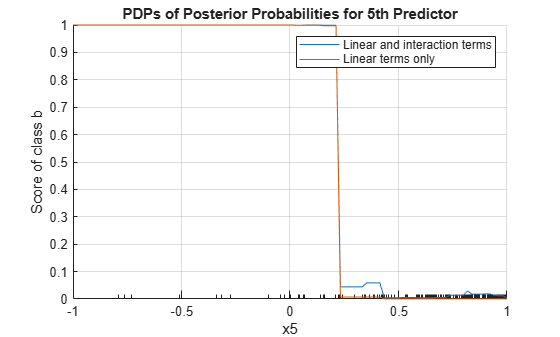The plot shows that the partial dependence of the scores (posterior probabilities) on `x5` varies depending on whether the model includes the interaction terms, especially where `x5` is between 0.2 and 0.45.

Train a support vector machine (SVM) regression model using the `carsmall` data set, and create a PDP for two predictor variables. Then, extract partial dependence estimates from the output of `plotPartialDependence`. Alternatively, you can get the partial dependence values by using the `partialDependence` function.

Load the `carsmall` data set.

`load carsmall`

Specify `Weight`, `Cylinders`, `Displacement`, and `Horsepower` as the predictor variables (`Tbl`).

`Tbl = table(Weight,Cylinders,Displacement,Horsepower);`

Construct an SVM regression model using `Tbl` and the response variable `MPG`. Use a Gaussian kernel function with an automatic kernel scale.

```Mdl = fitrsvm(Tbl,MPG,'ResponseName','MPG', ... 'CategoricalPredictors','Cylinders','Standardize',true, ... 'KernelFunction','gaussian','KernelScale','auto');```

Create a PDP that visualizes partial dependence of predicted responses (`MPG`) on the predictor variables `Weight` and `Cylinders`. Specify query points to compute the partial dependence for `Weight` by using the `'QueryPoints'` name-value pair argument. You cannot specify the `'QueryPoints'` value for `Cylinders` because it is a categorical variable. `plotPartialDependence` uses all categorical values.

```pt = linspace(min(Weight),max(Weight),50)'; ax = plotPartialDependence(Mdl,{'Weight','Cylinders'},'QueryPoints',{pt,[]}); view(140,30) % Modify the viewing angle```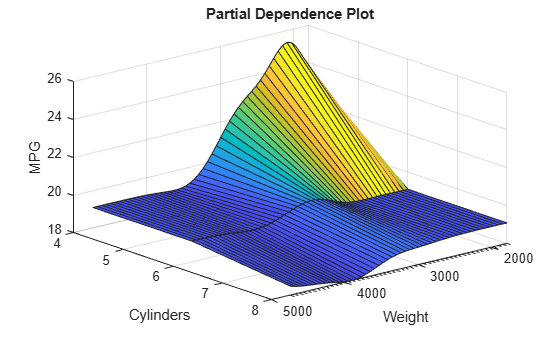The PDP shows an interaction effect between `Weight` and `Cylinders`. The partial dependence of `MPG` on `Weight` changes depending on the value of `Cylinders`.

Extract the estimated partial dependence of `MPG` on `Weight` and `Cylinders`. The `XData`, `YData`, and `ZData` values of `ax.Children` are x-axis values (the first selected predictor values), y-axis values (the second selected predictor values), and z-axis values (the corresponding partial dependence values), respectively.

```xval = ax.Children.XData; yval = ax.Children.YData; zval = ax.Children.ZData;```

Alternatively, you can get the partial dependence values by using the `partialDependence` function.

`[pd,x,y] = partialDependence(Mdl,{'Weight','Cylinders'},'QueryPoints',{pt,[]});`

`pd` contains the partial dependence values for the query points `x` and `y`.

If you specify `'Conditional'` as `'absolute'`, `plotPartialDependence` creates a figure including a PDP, a scatter plot, and a set of ICE plots. `ax.Children(1)` and `ax.Children(2)` correspond to the PDP and scatter plot, respectively. The remaining elements of `ax.Children` correspond to the ICE plots. The `XData` and `YData` values of `ax.Children(i)` are x-axis values (the selected predictor values) and y-axis values (the corresponding partial dependence values), respectively.

## Input Arguments

collapse all

Regression model, specified as a full or compact regression model object, as given in the following tables of supported models.

If `RegressionMdl` is a model object that does not contain predictor data (for example, a compact model), you must provide the input argument `Data`.

`plotPartialDependence` does not support a model object trained with a sparse matrix. When you train a model, use a full numeric matrix or table for predictor data where rows correspond to individual observations.

Classification model, specified as a full or compact classification model object, as given in the following tables of supported models.

ModelFull or Compact Model Object
Discriminant analysis classifier`ClassificationDiscriminant`, `CompactClassificationDiscriminant`
Multiclass model for support vector machines or other classifiers`ClassificationECOC`, `CompactClassificationECOC`
Ensemble of learners for classification`ClassificationEnsemble`, `CompactClassificationEnsemble`, `ClassificationBaggedEnsemble`
Generalized additive model (GAM)`ClassificationGAM`, `CompactClassificationGAM`
Gaussian kernel classification model using random feature expansion`ClassificationKernel`
k-nearest neighbor classifier`ClassificationKNN`
Linear classification model`ClassificationLinear`
Multiclass naive Bayes model`ClassificationNaiveBayes`, `CompactClassificationNaiveBayes`
Neural network classifier`ClassificationNeuralNetwork`, `CompactClassificationNeuralNetwork`
Support vector machine (SVM) classifier for one-class and binary classification`ClassificationSVM`, `CompactClassificationSVM`
Binary decision tree for multiclass classification`ClassificationTree`, `CompactClassificationTree`
Bagged ensemble of decision trees`TreeBagger`, `CompactTreeBagger`

If `ClassificationMdl` is a model object that does not contain predictor data (for example, a compact model), you must provide the input argument `Data`.

`plotPartialDependence` does not support a model object trained with a sparse matrix. When you train a model, use a full numeric matrix or table for predictor data where rows correspond to individual observations.

Predictor variables, specified as a vector of positive integers, character vector, string scalar, string array, or cell array of character vectors. You can specify one or two predictor variables, as shown in the following tables.

One Predictor Variable

ValueDescription
positive integerIndex value corresponding to the column of the predictor data.
character vector or string scalar

Name of a predictor variable. The name must match the entry in `RegressionMdl.PredictorNames` or `ClassificationMdl.PredictorNames`.

Two Predictor Variables

ValueDescription
vector of two positive integersIndex values corresponding to the columns of the predictor data.
string array or cell array of character vectors

Names of predictor variables. Each element in the array is the name of a predictor variable. The names must match the entries in `RegressionMdl.PredictorNames` or `ClassificationMdl.PredictorNames`.

Example: `{'x1','x3'}`

Data Types: `single` | `double` | `char` | `string` | `cell`

Class labels, specified as a categorical or character array, logical or numeric vector, or cell array of character vectors. The values and data types in `Labels` must match those of the class names in the `ClassNames` property of `ClassificationMdl` (`ClassificationMdl.ClassNames`).

This argument is valid only when you specify a classification model object `ClassificationMdl`.

Example: `{'red','blue'}`

Example: `ClassificationMdl.ClassNames([1 3])` specifies `Labels` as the first and third classes in `ClassificationMdl`.

Data Types: `single` | `double` | `logical` | `char` | `cell` | `categorical`

Predictor data, specified as a numeric matrix or table. Each row of `Data` corresponds to one observation, and each column corresponds to one variable.

`Data` must be consistent with the predictor data that trained the model (`RegressionMdl` or `ClassificationMdl`), stored in either the `X` or `Variables` property.

• If you trained the model using a numeric matrix, then `Data` must be a numeric matrix. The variables making up the columns of `Data` must have the same number and order as the predictor variables that trained the model.

• If you trained the model using a table (for example, `Tbl`), then `Data` must be a table. All predictor variables in `Data` must have the same variable names and data types as the names and types in `Tbl`. However, the column order of `Data` does not need to correspond to the column order of `Tbl`.

• `plotPartialDependence` does not support a sparse matrix.

If `RegressionMdl` or `ClassificationMdl` is a model object that does not contain predictor data, you must provide `Data`. If the model is a full model object that contains predictor data and you specify this argument, then `plotPartialDependence` does not use the predictor data in the model and uses `Data` only.

Data Types: `single` | `double` | `table`

### Name-Value Pair Arguments

Specify optional comma-separated pairs of `Name,Value` arguments. `Name` is the argument name and `Value` is the corresponding value. `Name` must appear inside quotes. You can specify several name and value pair arguments in any order as `Name1,Value1,...,NameN,ValueN`.

Example: `plotPartialDependence(Mdl,Vars,Data,'NumObservationsToSample',100,'UseParallel',true)` creates a PDP by using 100 sampled observations in `Data` and executing `for`-loop iterations in parallel.

Plot type, specified as `'none'`, `'absolute'`, or `'centered'`.

ValueDescription
`'none'`

`plotPartialDependence` creates a PDP. The plot type depends on the number of predictor variables specified in `Vars` and the number of class labels specified in `Labels` (for a classification model).

• One predictor variable and one class label — `plotPartialDependence` computes partial dependence at the query points, and creates a 2-D line plot of the partial dependence.

• One predictor variable and multiple class labels — `plotPartialDependence` creates one figure containing multiple 2-D line plots for the selected classes.

• Two predictor variables and one class label — `plotPartialDependence` creates a surface plot of partial dependence against the two variables.

`'absolute'`

`plotPartialDependence` creates a figure including the following three types of plots:

• PDP with a red line

• Scatter plot of the selected predictor variable and predicted responses or scores with circle markers

• ICE plot for each observation with a gray line

This value is valid when you select only one predictor variable in `Vars` and one class label in `Labels` (for a classification model).

`'centered'`

`plotPartialDependence` creates a figure including the same three types of plots as `'absolute'`. The function offsets plots so that all plots start from zero.

This value is valid when you select only one predictor variable in `Vars` and one class label in `Labels` (for a classification model).

Example: `'Conditional','absolute'`

Flag to include interaction terms of the generalized additive model (GAM) in the partial dependence computation, specified as `true` or `false`. This argument is valid only for a GAM. That is, you can specify this argument only when `RegressionMdl` is `RegressionGAM` or `CompactRegressionGAM`, or `ClassificationMdl` is `ClassificationGAM` or `CompactClassificationGAM`.

The default `'IncludeInteractions'` value is `true` if the model contains interaction terms. The value must be `false` if the model does not contain interaction terms.

Example: `'IncludeInteractions',false`

Data Types: `logical`

Flag to include an intercept term of the generalized additive model (GAM) in the partial dependence computation, specified as `true` or `false`. This argument is valid only for a GAM. That is, you can specify this argument only when `RegressionMdl` is `RegressionGAM` or `CompactRegressionGAM`, or `ClassificationMdl` is `ClassificationGAM` or `CompactClassificationGAM`.

Example: `'IncludeIntercept',false`

Data Types: `logical`

Number of observations to sample, specified as a positive integer. The default value is the number of total observations in `Data` or the model (`RegressionMdl` or `ClassificationMdl`). If you specify a value larger than the number of total observations, then `plotPartialDependence` uses all observations.

`plotPartialDependence` samples observations without replacement by using the `datasample` function and uses the sampled observations to compute partial dependence.

`plotPartialDependence` displays minor tick marks at the unique values of the sampled observations.

If you specify `'Conditional'` as either `'absolute'` or `'centered'`, `plotPartialDependence` creates a figure including an ICE plot for each sampled observation.

Example: `'NumObservationsToSample',100`

Data Types: `single` | `double`

Axes in which to plot, specified as an axes object. If you do not specify the axes and if the current axes are Cartesian, then `plotPartialDependence` uses the current axes (`gca`). If axes do not exist, `plotPartialDependence` plots in a new figure.

Example: `'Parent',ax`

Points to compute partial dependence for numeric predictors, specified as a numeric column vector, a numeric two-column matrix, or a cell array of two numeric column vectors.

• If you select one predictor variable in `Vars`, use a numeric column vector.

• If you select two predictor variables in `Vars`:

• Use a numeric two-column matrix to specify the same number of points for each predictor variable.

• Use a cell array of two numeric column vectors to specify a different number of points for each predictor variable.

The default value is a numeric column vector or a numeric two-column matrix, depending on the number of selected predictor variables. Each column contains 100 evenly spaced points between the minimum and maximum values of the sampled observations for the corresponding predictor variable.

If `'Conditional'` is `'absolute'` or `'centered'`, then the software adds the predictor data values (`Data` or predictor data in `RegressionMdl` or `ClassificationMdl`) of the selected predictors to the query points.

You cannot modify `'QueryPoints'` for a categorical variable. The `plotPartialDependence` function uses all categorical values in the selected variable.

If you select one numeric variable and one categorical variable, you can specify `'QueryPoints'` for a numeric variable by using a cell array consisting of a numeric column vector and an empty array.

Example: `'QueryPoints',{pt,[]}`

Data Types: `single` | `double` | `cell`

Flag to run in parallel, specified as `true` or `false`. If you specify `'UseParallel',true`, the `plotPartialDependence` function executes `for`-loop iterations in parallel by using `parfor` when predicting responses or scores for each observation and averaging them. This option requires Parallel Computing Toolbox™.

Example: `'UseParallel',true`

Data Types: `logical`

## Output Arguments

collapse all

Axes of the plot, returned as an axes object. For details on how to modify the appearance of the axes and extract data from plots, see Axes Appearance and Extract Partial Dependence Estimates from Plots.

## More About

collapse all

### Partial Dependence for Regression Models

Partial dependence represents the relationships between predictor variables and predicted responses in a trained regression model. `plotPartialDependence` computes the partial dependence of predicted responses on a subset of predictor variables by marginalizing over the other variables.

Consider partial dependence on a subset XS of the whole predictor variable set X = {x1, x2, …, xm}. A subset XS includes either one variable or two variables: XS = {xS1} or XS = {xS1, xS2}. Let XC be the complementary set of XS in X. A predicted response f(X) depends on all variables in X:

f(X) = f(XS, XC).

The partial dependence of predicted responses on XS is defined by the expectation of predicted responses with respect to XC:

`${f}^{S}\left({X}^{S}\right)={E}_{C}\left[f\left({X}^{S},{X}^{C}\right)\right]=\int f\left({X}^{S},{X}^{C}\right){p}_{C}\left({X}^{C}\right)d{X}^{C},$`

where pC(XC) is the marginal probability of XC, that is, ${p}_{C}\left({X}^{C}\right)\approx \int p\left({X}^{S},{X}^{C}\right)d{X}^{S}$. Assuming that each observation is equally likely, and the dependence between XS and XC and the interactions of XS and XC in responses is not strong, `plotPartialDependence` estimates the partial dependence by using observed predictor data as follows:

 ${f}^{S}\left({X}^{S}\right)\approx \frac{1}{N}\sum _{i=1}^{N}f\left({X}^{S},{X}_{i}{}^{C}\right),$ (1)

where N is the number of observations and Xi = (XiS, XiC) is the ith observation.

When you call the `plotPartialDependence` function, you can specify a trained model (f(·)) and select variables (XS) by using the input arguments `RegressionMdl` and `Vars`, respectively. `plotPartialDependence` computes the partial dependence at 100 evenly spaced points of XS or the points that you specify by using the `'QueryPoints'` name-value pair argument. You can specify the number (N) of observations to sample from given predictor data by using the `'NumObservationsToSample'` name-value pair argument.

### Individual Conditional Expectation for Regression Models

An individual conditional expectation (ICE) , as an extension of partial dependence, represents the relationship between a predictor variable and the predicted responses for each observation. While partial dependence shows the averaged relationship between predictor variables and predicted responses, a set of ICE plots disaggregates the averaged information and shows an individual dependence for each observation.

`plotPartialDependence` creates an ICE plot for each observation. A set of ICE plots is useful to investigate heterogeneities of partial dependence originating from different observations. `plotPartialDependence` can also create ICE plots with any predictor data provided through the input argument `Data`. You can use this feature to explore predicted response space.

Consider an ICE plot for a selected predictor variable xS with a given observation XiC, where XS = {xS}, XC is the complementary set of XS in the whole variable set X, and Xi = (XiS, XiC) is the ith observation. The ICE plot corresponds to the summand of the summation in Equation 1:

`${f}^{S}{}_{i}\left({X}^{S}\right)=f\left({X}^{S},{X}_{i}{}^{C}\right).$`

`plotPartialDependence` plots ${f}^{S}{}_{i}\left({X}^{S}\right)$ for each observation i when you specify `'Conditional'` as `'absolute'`. If you specify `'Conditional'` as `'centered'`, `plotPartialDependence` draws all plots after removing level effects due to different observations:

`${f}^{S}{}_{i,\text{centered}}\left({X}^{S}\right)=f\left({X}^{S},{X}_{i}{}^{C}\right)-f\left(\mathrm{min}\left({X}^{S}\right),{X}_{i}{}^{C}\right).$`

This subtraction ensures that each plot starts from zero, so that you can examine the cumulative effect of XS and the interactions between XS and XC.

### Partial Dependence and ICE for Classification Models

In the case of classification models, `plotPartialDependence` computes the partial dependence and individual conditional expectation in the same way as for regression models, with one exception: instead of using the predicted responses from the model, the function uses the predicted scores for the classes specified in `Labels`.

### Weighted Traversal Algorithm

The weighted traversal algorithm is a method to estimate partial dependence for a tree-based model. The estimated partial dependence is the weighted average of response or score values corresponding to the leaf nodes visited during the tree traversal.

Let XS be a subset of the whole variable set X and XC be the complementary set of XS in X. For each XS value to compute partial dependence, the algorithm traverses a tree from the root (beginning) node down to leaf (terminal) nodes and finds the weights of leaf nodes. The traversal starts by assigning a weight value of one at the root node. If a node splits by XS, the algorithm traverses to the appropriate child node depending on the XS value. The weight of the child node becomes the same value as its parent node. If a node splits by XC, the algorithm traverses to both child nodes. The weight of each child node becomes a value of its parent node multiplied by the fraction of observations corresponding to each child node. After completing the tree traversal, the algorithm computes the weighted average by using the assigned weights.

For an ensemble of bagged trees, the estimated partial dependence is an average of the weighted averages over the individual trees.

## Algorithms

`plotPartialDependence` uses a `predict` function to predict responses or scores. `plotPartialDependence` chooses the proper `predict` function according to the model (`RegressionMdl` or `ClassificationMdl`) and runs `predict` with its default settings. For details about each `predict` function, see the `predict` functions in the following two tables. If the specified model is a tree-based model (not including a boosted ensemble of trees) and `'Conditional'` is `'none'`, then `plotPartialDependence` uses the weighted traversal algorithm instead of the `predict` function. For details, see Weighted Traversal Algorithm.

Regression Model Object

Model TypeFull or Compact Regression Model ObjectFunction to Predict Responses
Bootstrap aggregation for ensemble of decision trees`CompactTreeBagger``predict`
Bootstrap aggregation for ensemble of decision trees`TreeBagger``predict`
Ensemble of regression models`RegressionEnsemble`, `RegressionBaggedEnsemble`, `CompactRegressionEnsemble``predict`
Gaussian kernel regression model using random feature expansion`RegressionKernel``predict`
Gaussian process regression`RegressionGP`, `CompactRegressionGP``predict`
Generalized additive model`RegressionGAM`, `CompactRegressionGAM``predict`
Generalized linear mixed-effect model`GeneralizedLinearMixedModel``predict`
Generalized linear model`GeneralizedLinearModel`, `CompactGeneralizedLinearModel``predict`
Linear mixed-effect model`LinearMixedModel``predict`
Linear regression`LinearModel`, `CompactLinearModel``predict`
Linear regression for high-dimensional data`RegressionLinear``predict`
Neural network regression model`RegressionNeuralNetwork`, `CompactRegressionNeuralNetwork``predict`
Nonlinear regression`NonLinearModel``predict`
Regression tree`RegressionTree`, `CompactRegressionTree``predict`
Support vector machine`RegressionSVM`, `CompactRegressionSVM``predict`

Classification Model Object

Model TypeFull or Compact Classification Model ObjectFunction to Predict Labels and Scores
Discriminant analysis classifier`ClassificationDiscriminant`, `CompactClassificationDiscriminant``predict`
Multiclass model for support vector machines or other classifiers`ClassificationECOC`, `CompactClassificationECOC``predict`
Ensemble of learners for classification`ClassificationEnsemble`, `CompactClassificationEnsemble`, `ClassificationBaggedEnsemble``predict`
Gaussian kernel classification model using random feature expansion`ClassificationKernel``predict`
Generalized additive model`ClassificationGAM`, `CompactClassificationGAM``predict`
k-nearest neighbor model`ClassificationKNN``predict`
Linear classification model`ClassificationLinear``predict`
Naive Bayes model`ClassificationNaiveBayes`, `CompactClassificationNaiveBayes``predict`
Neural network classifier`ClassificationNeuralNetwork`, `CompactClassificationNeuralNetwork``predict`
Support vector machine for one-class and binary classification`ClassificationSVM`, `CompactClassificationSVM``predict`
Binary decision tree for multiclass classification`ClassificationTree`, `CompactClassificationTree``predict`
Bagged ensemble of decision trees`TreeBagger`, `CompactTreeBagger``predict`

## Alternative Functionality

• `partialDependence` computes partial dependence without visualization. The function can compute partial dependence for two variables and multiple classes in one function call.

 Friedman, Jerome. H. “Greedy Function Approximation: A Gradient Boosting Machine.” The Annals of Statistics 29, no. 5 (2001): 1189-1232.

 Goldstein, Alex, Adam Kapelner, Justin Bleich, and Emil Pitkin. “Peeking Inside the Black Box: Visualizing Statistical Learning with Plots of Individual Conditional Expectation.” Journal of Computational and Graphical Statistics 24, no. 1 (January 2, 2015): 44–65.

 Hastie, Trevor, Robert Tibshirani, and Jerome Friedman. The Elements of Statistical Learning. New York, NY: Springer New York, 2001.

Download ebook